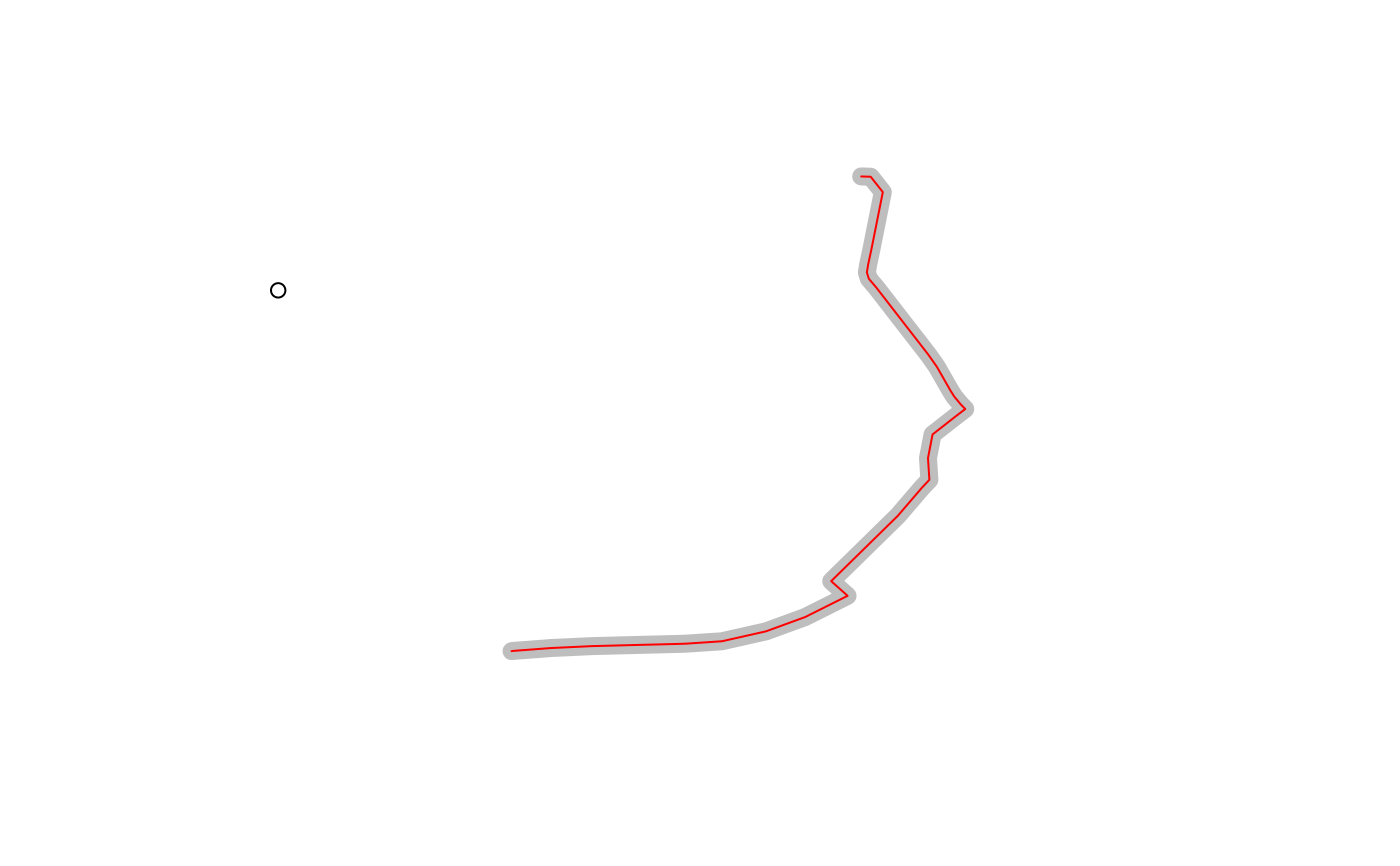Split route in two at point on or near network

## Usage

``route_split(r, p)``

## Arguments

r

An `sf` object with one feature containing a linestring geometry to be split

p

A point represented by an `sf` object the will split the `route`

## Value

An sf object with 2 feature

## Examples

``````sample_routes <- routes_fast_sf[2:6, NULL]
r <- sample_routes[2, ]
p <- sf::st_sfc(sf::st_point(c(-1.540, 53.826)), crs = sf::st_crs(r))
plot(r\$geometry, lwd = 9, col = "grey")